There’s one topic that I repeatedly find myself drawn to – the relative better-ness of axial and radial-flux electric motors.

Now, there are of course plenty of comparison papers out, I’ve made a few blog posts myself in the past, and real-world applicability also depends on nasty sidelines stuff like manufacturability and being able to convince both investors and OEMs of your solution.

So, what I decided to do here is a super-barebones first-principles comparison of an axial-flux PM motor to a radial-flux outrunner.

Why outrunner, you might ask? Well, because I’m pretty sure AFM would beat an inrunner in the kind of super-simple analysis that I’m about to do, and that would be just boring. And, my personal experience is that super-simple analysis doesn’t actually work that well for inrunners – for instance blindly optimizing the split ratio (inner/outer diameter) to maximize the torque would lead to values like 0.33, leading to problems with saturation and thermal management.

So, that’s why outrunners. But what about the analysis itself?

### How bare-bones are we talking about

The analysis here aims to be as simple as possible. We’ll be only looking at the “interesting” part of the geometry, i.e. the surroundings of the airgap where all the magic happens. We won’t be looking at any kind of losses (Key limitation #1), nor will we be considering saturation or non-ideal flux paths (Key limitation #2), let alone manufacturability or anything like that (strike #3).

Instead, we’ll be looking at only a few principles and assumptions:

• Outer diameter of the stator core is fixed
• Both machines will have the same core length in the axial direction
• We’ll only allow a certain flux density in the stator teeth, assumed to only contain the working PM flux (so no armature reaction or leakage fluxes)
• We’ll optimize (on paper) the width of the stator teeth (at their narrowest) point
• For the axial-flux machine, we’ll also optimize the inner diameter.

For the torque production, we’ll again be using the Lorentz formula = flux density x current x moment arm. As it will turn out, each of these numbers will depend on the slot and tooth geometry, as we’ll soon see.

Now, let’s do it.

## Axial Flux Principles

Below, you can see my superior MS Paint illustration of the airgap-facing side of an AFM stator. The grey blocks are the stator teeth, while the red thingy represents the winding (double-layer concentrated in out case).

You can see the interesting radii marked with the black lines, and also some vectors. Indeed, the useful current density flow radially, flux density is axial, resulting in force in the circumferential direction, resulting in useful torque.

### Main equations

The main assumptions / governing equations are listed below:

• The slot width is equal to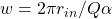, where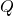is the number of slots and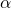is the relative slot pitch. This is a key number – the number of slots will cancel itself out.
• Correspondingly, the tooth width at the inner radius is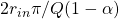• Correspondingly, the airgap flux density is proportional to the allowed tooth flux density: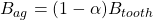.
• The total area of the slot, perpendicular to the current density is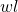. The total slot-bound current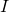is proportional to this number.

The torque is then obtained from the Lorentz force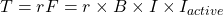. For an axial-flux machine, the active length in this case is between the inner and outer radii, while the moment arm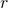is the average airgap radius. Thus, we get for the torque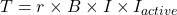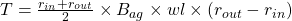With a little mathematics, we can conclude two things:

• For any arbitrary slot pitch, the optimal inner radius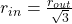• Regardless of the inner radius, the optimal slot pitch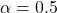, meaning the slots are as wide as the teeth in their narrowest point

Now, let’s do the same exercise for an outrunner.

Again, you can find a beautific illustration below. You can see two teeth, the red winding volume between them (no end-winding shown in this case), along with some dimensions and vectors.

### Main equations

Similar reasoning can be applied to an radial-flux outrunner topology:

• The tooth width can now be obtained from the outer radius and the slot pitch: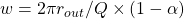• The airgap flux density is again.
• The slot depth is maximized by requiring that the slot bottom width is zero, i.e. that the tooth sides meet each other at the slot bottom. Exact solution would require some trigonometry, but a good-enough approximation can be found from circle arcs: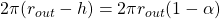, yielding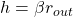.
• The total slot area (all slots combined) can be obtained from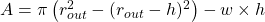.

The torque is then again proportional to the slot area (via the slot-bound current), the moment arm (in this case the outer radius), the flux density, and the active length of the machine: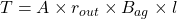This time, we are left with one variable to optimize; namely the slot pitch. After all, it influences the slot area (via the slot depth and tooth width both), and then the airgap flux density.

In any case, by expanding the expression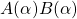, and then setting its derivative to zero yields us two extrema: one trivial at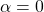and the useful one with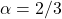. (Actually doing this math is tedious and very high-school-ish.)

In other words, the optimal design has quite narrow teeth.

## Showdown

So, what about the results then?

Luckily, it turns out that we don’t really have to fix any design constraints to compare the relative torque performance of an axial flux motor to an outrunner. Indeed, all of our free variables – the outer radius, tooth flux density, slot current density and fill factor, and the axial length – they all get cancelled out.

In the end, this simple analysis presented predicts roughly 53.96 % larger torques for the outrunner.

I got a little lazy in the end and did not simplify the final torque expressions (Matlab to the resque), but I’m sure there’s a nice expression with lots of square roots behind that number.

### Verification

A post would be nothing without an EMDtool verification, right?

Well, below you can see the torque curve of an outrunner motor compared to an AFM, both designed according to the principles presented here. As can be seen, at low current densities the results are indeed close to what expected…

…until reality hits you in the face: see some “minor” saturation in the outrunner below.

So, again a nice example on the limitations of simple analytical methods 😁

Check out EMDtool - Electric Motor Design toolbox for Matlab.

Need help with electric motor design or design software? Let's get in touch - satisfaction guaranteed!Axial versus Radial Flux – First Principles